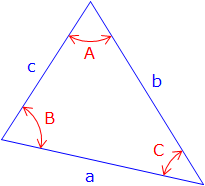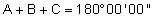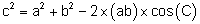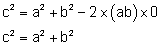### 2. Plane triangle formulae

A plane triangle, Figure C-2, has six parts: three angles and three sides.Figure C-2 Plane triangle

Individual angles may be acute (<90°), right (=90°), or obtuse (>90°) with their sum exactly 180°.

To geometrically define a triangle requires three parts, including at least one side, be fixed. Why a side? Because fixing angles alone does not constrain the size of the triangle. The two triangles in Figure C-3 are different sizes even though they have identical angles.Figure C-3 Same angles; different triangle sizes

Equations for triangle trigonometry are:

 Angle condition:Equation C-1 Law of Sines:Equation C-2 Law of Cosines:Equation C-3

A right triangle is a special case where one angle is exactly 90°. Applying the Law of Cosines, Equation C-3, to a right triangle, Figure C-4:Figure C-4 Right triangleBecause cos(90°) = 0The Law of Cosines becomes the Pythagorean Theorem.

Depending on which parts of it are known the area of a triangle can be determined using one of two equations:

 Using two sides and an included angle:Equation C-4 Using three sides:Equation C-5 where:Equation C-6